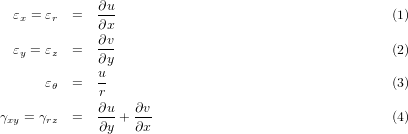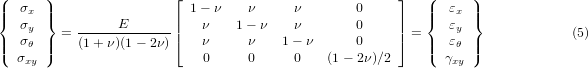#### 2.7 Axisymmetric Elements

Implementation relies on elements located exclusively in x,y plane. The coordinate x corresponds to radius, y is the axis of rotation. Approximation of displacement functions u,v is carried out on a particular finite element. Nonzero strains readStress components can be computed from elasticity matrix. Note that this matrix corresponds to a submatrix of the full 3D elasticity matrix.In OOFEM, the strain vector is arranged as {εxyθ,0,0xy}T and the stress vector {σxyθ,0,0xy}T. Implementation assumes a segment of 1 rad.

##### 2.7.1 Axisymm3d element

Implementation of triangular three-node finite element for axisymmetric continuum. Each node has 2 degrees of freedom. Node numbering and edge position is the same as in Fig. 7. The element features are summarized in Table 30.

 Keyword Axisymm3d Description Triangular axisymmetric linear element Specific parameters [NIP #(in)] [NIPfish #(in)] Parameters NIP: allows to set the number of integration points (possible completions are 1 (default), 4 and 7 point integration rule). Unknowns Two dofs (u-displacement, v-displacement) are required in each node. Approximation Linear approximation of displacement and geometry. Integration The integration can be altered using NIP paramter (default is 1 point integration). Features - CS properties - Loads Boundary and body loads are supported. Nlgeo 0. Status -

Table 30: Axisymm3d element summary

##### 2.7.2 Q4axisymm element

Implementation of quadratic isoparametric eight-node quadrilateral - finite element for axisymmetric 3d continuum. Each node has 2 degrees of freedom. The element features are summarized in Table 31.

 Keyword Q4axisymm Description Quadratic isoparametric eight-node quadrilateral for axisymmetric analysis Specific parameters [NIP #(in)] [NIPfish #(in)] Parameters NIP: allows to set the number of integration points for integration of terms corresponding to εx and εy strains (possible completions are 1, 4 (default), 9, and 16). NIPfish: allows to set the number of integration points for integration of remain terms (corresponding to εθ and γrz) (Supported values include 1 (default), 4, 9, and 16 integration point formula). Unknowns Two dofs (u-displacement, v-displacement) are required in each node. Approximation Quadratic approximation of displacement and geometry. Integration The integration of terms corresponding to εx and εy strains can be altered using NIP parameter (default is 4 point formula). The remaining terms (creesponding to εθ and γrz) are integrated by default using 1 point formula (see NIPfish parameter). Features - CS properties - Loads No boundary and body loads are supported. Nlgeo 0. Status -

Table 31: Q4axisymm element summary

##### 2.7.3 L4axisymm element

Implementation of isoparametric four-node quadrilateral axisymmetric finite element with linear interpolations of displacements u,v. Node numbering and edge position is the same as in Fig. 5. The element features are summarized in Table 32.

 Keyword L4axisymm Description Isoparametric four-node quadrilateral element for axisymmetric analysis Specific parameters [NIP #(in)] Parameters NIP: allows to set the number of integration points for integration of terms corresponding to εx and εy strains (possible completions are 1, 4 (default), 9, and 16). Unknowns Two dofs (u-displacement, v-displacement) are required in each node. Approximation Linear approximation of displacement and geometry. Integration The integration of εx and εy strains can be altered using NIP parameter (possible completions are 1, 4 (default), 9 or 16 point integration rule). The remaining strain components (εθ and γrz) are integrated using one point integration formula. Features - CS properties - Loads Boundary and body loads supported. Nlgeo 0. Status -

Table 32: L4axisymm element summary